# HSSlive: Plus One & Plus Two Notes & Solutions for Kerala State Board

## AP Board Class 7 Maths Chapter 4 Lines and Angles Ex 7 Textbook Solutions PDF: Download Andhra Pradesh Board STD 7th Maths Chapter 4 Lines and Angles Ex 7 Book AnswersAP Board Class 7 Maths Chapter 4 Lines and Angles Ex 7 Textbook Solutions PDF: Download Andhra Pradesh Board STD 7th Maths Chapter 4 Lines and Angles Ex 7 Book Answers

## Andhra Pradesh State Board Class 7th Maths Chapter 4 Lines and Angles Ex 7 Books Solutions

 Board AP Board Materials Textbook Solutions/Guide Format DOC/PDF Class 7th Subject Maths Chapters Maths Chapter 4 Lines and Angles Ex 7 Provider Hsslive

2. Click on the Andhra Pradesh Board Class 7th Maths Chapter 4 Lines and Angles Ex 7 Answers.
3. Look for your Andhra Pradesh Board STD 7th Maths Chapter 4 Lines and Angles Ex 7 Textbooks PDF.
4. Now download or read the Andhra Pradesh Board Class 7th Maths Chapter 4 Lines and Angles Ex 7 Textbook Solutions for PDF Free.

## AP Board Class 7th Maths Chapter 4 Lines and Angles Ex 7 Textbooks Solutions with Answer PDF Download

Find below the list of all AP Board Class 7th Maths Chapter 4 Lines and Angles Ex 7 Textbook Solutions for PDF’s for you to download and prepare for the upcoming exams:

Question 1.
Fill up the blanks
(i) The line which intersects two or more lines at distinct points is called __________
(ii) If the pair of alternate interior angles are equal then the lines are ______________
(iii) The sum of interior angles on the same side of the transversal are supplementary then the lines are ____________
(iv) If two lines intersect each other then the number of common points they have
Solution:
i) The line which intersects two or more lines at distinct points is called transversal.
ii) If the pairs of alternate interior angles are equal then the lines are parallel.
ii) The sum of interior angles on the same side of the transversal are supplementary then the lines are parallel.
iv) If two lines intersect each other then the number of common points they have Only one.

Question 2.
In the adjacent figure, the lines ‘P and ‘m’ are parallel and ‘n’ is a transversal. Fill in the blanks for all the situations given below.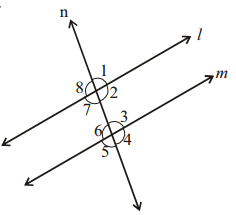(i) If ∠1 = 80° then∠2 =
(ii) If ∠3 = 45° then ∠7 =
(iii) If ∠2 = 90° then ∠8 =
(iv) If ∠4 = 100° then ∠8 =
Solution:
(i) If ∠1 = 80° then∠2 = 100
(ii) If ∠3 = 45° then ∠7 = 45
(iii) If ∠2 = 90° then ∠8 = 90
(iv) If ∠4 = 100° then ∠8 = 100

Question 3.
Find the measures of x,y and z in the figure, where l || BC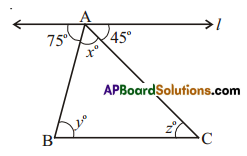Solution:
∠y = 75° (alt. tnt. angles)
∠z = 45° (ait. tnt. angles)
∠x + ∠y + ∠z = 180° (∵ int. angles of a triangle = 1800)
75° + 45° + ∠x = 180°
∠x = 180°- 120°
= 60°

Question 4.
ABCD is a quadrilateral in which AB || DC and AD || BC. Find ∠b, ∠c and ∠d.
Solution:
∠b + ∠50° = 180° (int. angles on the same side of AB)
∠b = 180° – 50° = 130°
Similarly ∠b + ∠c = ∠c + ∠d = ∠d + ∠50 = 180°
∠130° + ∠c = 180°
∠c = 180° – 130° = 50°
Also ∠c + ∠d = 50° +∠d = 180°
∠d = 180° – 50° = 130°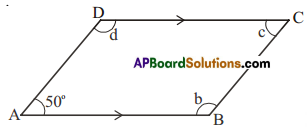Question 5.
In a given figure, ‘l’ and m’ are intersected by a transversal ‘n’. Is 1 || m?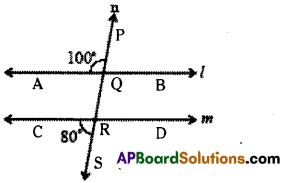Solution:
∠AQP and ∠CRS are exterior angles on the same side of the transversal n and their sum
100° + 80° = 180°
Hence l//m.

Question 6.
Find ∠a, ∠b, ∠c, ∠d and ∠e in the figure? Give reasons.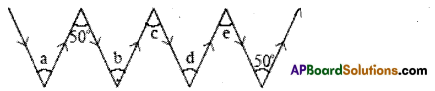Note: Two anow marks pointing in the same direction represent parallel lines.
Solution:
∠a = ∠50° (∵ alt. int, angle between two parallel lines)
∠b = 50° (∵ alt. int, angle between two parallel lines)
∠b = ∠c = 50° (∵ alt. int. angles between two parallel lines)
∠c = ∠d = 50° ( ∵ alt. int angles between two parallel lines)
∠e = ∠d = 50°(∵ alt. int. angles between two parallel lines)

## Andhra Pradesh Board Class 7th Maths Chapter 4 Lines and Angles Ex 7 Textbooks for Exam Preparations

Andhra Pradesh Board Class 7th Maths Chapter 4 Lines and Angles Ex 7 Textbook Solutions can be of great help in your Andhra Pradesh Board Class 7th Maths Chapter 4 Lines and Angles Ex 7 exam preparation. The AP Board STD 7th Maths Chapter 4 Lines and Angles Ex 7 Textbooks study material, used with the English medium textbooks, can help you complete the entire Class 7th Maths Chapter 4 Lines and Angles Ex 7 Books State Board syllabus with maximum efficiency.

## FAQs Regarding Andhra Pradesh Board Class 7th Maths Chapter 4 Lines and Angles Ex 7 Textbook Solutions

#### Can we get a Andhra Pradesh State Board Book PDF for all Classes?

Yes you can get Andhra Pradesh Board Text Book PDF for all classes using the links provided in the above article.

## Important Terms

Andhra Pradesh Board Class 7th Maths Chapter 4 Lines and Angles Ex 7, AP Board Class 7th Maths Chapter 4 Lines and Angles Ex 7 Textbooks, Andhra Pradesh State Board Class 7th Maths Chapter 4 Lines and Angles Ex 7, Andhra Pradesh State Board Class 7th Maths Chapter 4 Lines and Angles Ex 7 Textbook solutions, AP Board Class 7th Maths Chapter 4 Lines and Angles Ex 7 Textbooks Solutions, Andhra Pradesh Board STD 7th Maths Chapter 4 Lines and Angles Ex 7, AP Board STD 7th Maths Chapter 4 Lines and Angles Ex 7 Textbooks, Andhra Pradesh State Board STD 7th Maths Chapter 4 Lines and Angles Ex 7, Andhra Pradesh State Board STD 7th Maths Chapter 4 Lines and Angles Ex 7 Textbook solutions, AP Board STD 7th Maths Chapter 4 Lines and Angles Ex 7 Textbooks Solutions,
Share: